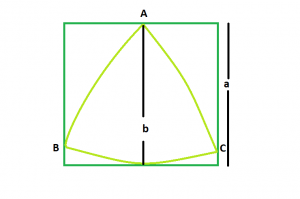Related Articles

# Biggest Reuleaux Triangle within A Square

• Last Updated : 11 Jun, 2021

Given an integer a which is the side of a square, the task is to find the biggest Reuleaux Triangle that can be inscribed within it.Examples:

Input: a = 6
Output: 25.3717

Input: a = 8
Output: 45.1053

Approach: We know that the Area of Reuleaux Triangle is 0.70477 * b2 where b is the distance between the parallel lines supporting the Reuleaux Triangle.
From the figure, it is clear that distance between parallel lines supporting the Reuleaux Triangle = Side of the square i.e. a
So, Area of the Reuleaux Triangle, A = 0.70477 * a2

Below is the implementation of the above approach:

## C++

 `// C++ Program to find the area``// of the biggest Reuleaux triangle``// that can be inscribed within a square``#include ``using` `namespace` `std;` `// Function to find the Area``// of the Reuleaux triangle``float` `ReuleauxArea(``float` `a)``{` `    ``// Side cannot be negative``    ``if` `(a < 0)``        ``return` `-1;` `    ``// Area of the Reuleaux triangle``    ``float` `A = 0.70477 * ``pow``(a, 2);``    ``return` `A;``}` `// Driver code``int` `main()``{``    ``float` `a = 6;``    ``cout << ReuleauxArea(a) << endl;``    ``return` `0;``}`

## Java

 `// Java Program to find the area``// of the biggest Reuleaux triangle``// that can be inscribed within a square``import` `java.lang.Math;``class` `cfg``{``// Function to find the Area``// of the Reuleaux triangle`` ``static` `double` `ReuleauxArea(``double` `a)``{` `    ``// Side cannot be negative``    ``if` `(a < ``0``)``        ``return` `-``1``;` `    ``// Area of the Reuleaux triangle``    ``double` `A = ``0.70477` `* Math.pow(a, ``2``);``    ``return` `A;``}` `// Driver code``public` `static` `void` `main(String[] args)``{``    ``double` `a= ``6``;``    ``System.out.println(ReuleauxArea(a) );``    ` `}``}``//This code is contributed by Mukul Singh.`

## Python3

 `# Python3 Program to find the area``# of the biggest Reuleaux triangle``# that can be inscribed within a square` `# Function to find the Area``# of the Reuleaux triangle``def` `ReuleauxArea(a) :` `    ``# Side cannot be negative``    ``if` `(a < ``0``) :``        ``return` `-``1` `    ``# Area of the Reuleaux triangle``    ``A ``=` `0.70477` `*` `pow``(a, ``2``);``    ``return` `A` `# Driver code``if` `__name__ ``=``=` `"__main__"` `:` `    ``a ``=` `6``    ``print``(ReuleauxArea(a))` `# This code is contributed by Ryuga`

## C#

 `// C# program to find area of the``//biggest  Reuleaux triangle that can be inscribed``//within a square``using` `System;``  ` `class` `GFG {``  ` `    ``// Function to find the area``    ``// of the reuleaux triangle``    ``static` `double` `reuleauxArea(``double` `a)``    ``{``  ` `     ``//Side cannot be negative``     ``if` `(a<0)``        ``return` `-1;``        ` `        ``// Area of the reauleaux triangle``        ``double` `A=0.70477*Math.Pow(a,2);``        ``return` `A;``    ``}``  ` `    ``// Driver code``    ``static` `public` `void` `Main()``    ``{``        ``double` `a= 6;``        ``Console.WriteLine(reuleauxArea( a));``    ``}``}``//This code is contributed by Mohit kumar 29`

## PHP

 ``

## Javascript

 ``
Output:
`25.3717`

Attention reader! Don’t stop learning now. Get hold of all the important DSA concepts with the DSA Self Paced Course at a student-friendly price and become industry ready.  To complete your preparation from learning a language to DS Algo and many more,  please refer Complete Interview Preparation Course.

In case you wish to attend live classes with experts, please refer DSA Live Classes for Working Professionals and Competitive Programming Live for Students.

My Personal Notes arrow_drop_up